# Group cohomology of groups of order 32

This article gives specific information, namely, group cohomology, about a family of groups, namely: groups of order 32.
View group cohomology of group families | View group cohomology of groups of a particular order |View other specific information about groups of order 32

This page describes the theory behind the homology and cohomology groups of groups of order 32.

View these in a broader context: group cohomology of groups of prime-fifth order | group cohomology of groups of order 2^n
Group Second part of GAP ID (GAP ID is (32,second part)) Hall-Senior number (among groups of order 32) Hall-Senior symbol Nilpotency class Group cohomology page
Cyclic group:Z32 1 7$(5)$ 1 See group cohomology of finite cyclic groups
SmallGroup(32,2) 2 18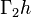$\Gamma_2h$ 2
Direct product of Z8 and Z4 3 5$(32)$ 1 See group cohomology of finite abelian groups
Semidirect product of Z8 and Z4 of M-type 4 19$\Gamma_2i$ 2
SmallGroup(32,5) 5 20$\Gamma_2j_1$ 2
Faithful semidirect product of E8 and Z4 6 46$\Gamma_7a_1$ 3
SmallGroup(32,7) 7 47$\Gamma_7a_2$ 3
SmallGroup(32,8) 8 48$\Gamma_7a_3$ 3
SmallGroup(32,9) 9 27$\Gamma_3c_1$ 3
SmallGroup(32,10) 10 28$\Gamma_3c_2$ 3
Wreath product of Z4 and Z2 11 31$\Gamma_3e$ 3
SmallGroup(32,12) 12 21$\Gamma_2j_2$ 2
Semidirect product of Z8 and Z4 of semidihedral type 13 30$\Gamma_3d_2$ 3
Semidirect product of Z8 and Z4 of dihedral type 14 29$\Gamma_3d_1$ 3
SmallGroup(32,15) 15 32$\Gamma_3f$ 3
Direct product of Z16 and Z2 16 6$(41)$ 1 See group cohomology of finite abelian groups
M32 17 22$\Gamma_2k$ 2
Dihedral group:D32 18 49$\Gamma_8a_1$ 4 See group cohomology of dihedral groups
Semidihedral group:SD32 19 50$\Gamma_8a_2$ 4 See group cohomology of semidihedral groups
Generalized quaternion group:Q32 20 51$\Gamma_8a_3$ 4 See group cohomology of dicyclic groups
Direct product of Z4 and Z4 and Z2 21 3$(2^21)$ 1
Direct product of SmallGroup(16,3) and Z2 22 11$\Gamma_2c_1$ 2
Direct product of SmallGroup(16,4) and Z2 23 12$\Gamma_2c_2$ 2
SmallGroup(32,24) 24 16$\Gamma_2f$ 2
Direct product of D8 and Z4 25 14$\Gamma_2e_1$ 2
Direct product of Q8 and Z4 26 15$\Gamma_2e_2$ 2
SmallGroup(32,27) 27 33$\Gamma_4a_1$ 2
SmallGroup(32,28) 28 36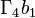$\Gamma_4b_1$ 2
SmallGroup(32,29) 29 37$\Gamma_4b_2$ 2
SmallGroup(32,30) 30 38$\Gamma_4c_1$ 2
SmallGroup(32,31) 31 39$\Gamma_4c_2$ 2
SmallGroup(32,32) 32 40$\Gamma_4c_3$ 2
SmallGroup(32,33) 33 41$\Gamma_4d$ 2
Generalized dihedral group for direct product of Z4 and Z4 34 34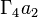$\Gamma_4a_2$ 2 See group cohomology of generalized dihedral groups
SmallGroup(32,35) 35 35$\Gamma_4a_3$ 2
Direct product of Z8 and V4 36 4$(31^2)$ 1 See group cohomology of finite abelian groups
Direct product of M16 and Z2 37 13$\Gamma_2d$ 2
Central product of D8 and Z8 38 17$\Gamma_2g$ 2
Direct product of D16 and Z2 39 23$\Gamma_3a_1$ 3
Direct product of SD16 and Z2 40 24$\Gamma_3a_2$ 3
Direct product of Q16 and Z2 41 25$\Gamma_3a_3$ 3
Central product of D16 and Z4 42 26$\Gamma_3b$ 3
Holomorph of Z8 43 44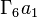$\Gamma_6a_1$ 3
SmallGroup(32,44) 44 45$\Gamma_6a_2$ 3
Direct product of E8 and Z4 45 2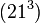$(21^3)$ 1 See group cohomology of finite abelian groups
Direct product of D8 and V4 46 8$\Gamma_2a_1$ 2
Direct product of Q8 and V4 47 9$\Gamma_2a_2$ 2
Direct product of SmallGroup(16,13) and Z2 48 10$\Gamma_2b$ 2
Inner holomorph of D8 49 42$\Gamma_5a_1$ 2 See group cohomology of extraspecial groups
Central product of D8 and Q8 50 43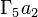$\Gamma_5a_2$ 2 See group cohomology of extraspecial groups
Elementary abelian group:E32 51 1$(1^5)$ 1 See group cohomology of elementary abelian groups